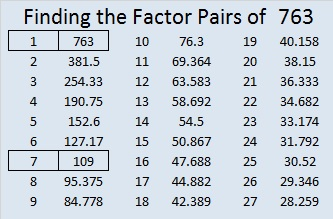# 763 and Level 3

It’s obvious that 763 is divisible by 7 so it is a composite number.

• 763 is a composite number.
• Prime factorization: 763 = 7 x 109
• The exponents in the prime factorization are 1 and 1. Adding one to each and multiplying we get (1 + 1)(1 + 1) = 2 x 2 = 4. Therefore 763 has exactly 4 factors.
• Factors of 763: 1, 7, 109, 763
• Factor pairs: 763 = 1 x 763 or 7 x 109
• 763 has no square factors that allow its square root to be simplified. √763 ≈ 27.6224546.Now try solving today’s puzzle:Print the puzzles or type the solution on this excel file: 10 Factors 2016-02-04

—————————————

Here’s a little more about the number 763:

763 is the sum of consecutive numbers three different ways:

• 381 + 382 = 763; that’s 2 consecutive numbers.
• 106 + 107 + 108 + 109 + 110 + 111 + 112 = 763; that’s 7 consecutive numbers.
• 48 + 49 + 50 + 51 + 52 + 53 + 54 + 55 + 56 + 57 + 58 + 59 + 60 + 61 = 763; that’s 14 consecutive numbers.

763 is also the sum of consecutive prime numbers two different ways:

• 67 + 71 + 73 + 79 + 83 + 89 + 97 + 101 + 103 = 763; that’s 9 consecutive primes.
• 13 + 17 + 19 + 23 + 29 + 31 + 37 + 41 + 43 + 47 + 53 + 59 + 61 + 67 + 71 + 73 + 79 = 763; that’s 17 consecutive primes.

763 is the hypotenuse of a Pythagorean triple, and 420² + 637² = 763².

763 is also the sum of three squares two different ways:

• 27² + 5² + 3² = 763
• 23² + 15² + 3² = 763

763^4 = 338,920,744,561, a number in which every digit appears at least one time. OEIS.org informs us 763 is the smallest number whose 4th power can make that claim.

—————————————This site uses Akismet to reduce spam. Learn how your comment data is processed.